Courses

# JEE Advanced Mock Test - 3

## 54 Questions MCQ Test Mock Test Series for JEE Main & Advanced 2021 | JEE Advanced Mock Test - 3

Description
This mock test of JEE Advanced Mock Test - 3 for JEE helps you for every JEE entrance exam. This contains 54 Multiple Choice Questions for JEE JEE Advanced Mock Test - 3 (mcq) to study with solutions a complete question bank. The solved questions answers in this JEE Advanced Mock Test - 3 quiz give you a good mix of easy questions and tough questions. JEE students definitely take this JEE Advanced Mock Test - 3 exercise for a better result in the exam. You can find other JEE Advanced Mock Test - 3 extra questions, long questions & short questions for JEE on EduRev as well by searching above.
QUESTION: 1

Solution:
QUESTION: 2

Solution:
QUESTION: 3

### A particle of charge q and mass m is projected with a velocity v0 towards a circular region having uniform magnetic field B perpendicular and into the plane of paper from point P as shown in the figure. R is the radius and O is the centre of the circular region. If the line OP makes an angle θ with the direction of v0 then the value of v0 so that particle passes through O is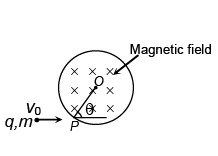Solution:
QUESTION: 4

A ray of light from a liquid ( μ = √3 ) is incident on a system of two right angled prism of refractive indices √3 and √2 as shown. The ray suffers zero deviation when emerges into air from CD. The angle of incidence i is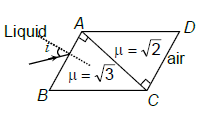Solution:
*Multiple options can be correct
QUESTION: 5

This section contains 2 multiple choice questions. Each question has 4 choices (A), (B), (C) and (D) for its
answer, out of which ONE OR MORE is/are correct

Two blocks A and B each of mass 1/2 kg is connected by a massless inextensible string and kept on horizontal surface. Coefficient of friction between block and surface is shown in figure. A force F = kt (where k = 1 N/s and t is time in second) applied on A. Then (g = 10 m/s2)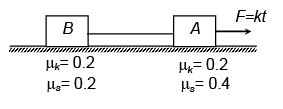Solution:
*Multiple options can be correct
QUESTION: 6

A 4 μF capacitor is given 20 μC charge and is connected with an uncharged capacitor ofcapacitance 2 μF as shown in figure. When switch S is closed.

Solution: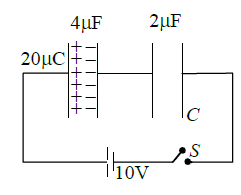QUESTION: 7

This section contains 2 paragraphs. Based upon one of paragraph 2 multiple choice questions and based
on the other paragraphs 3 multiple choice questions have to be answered. Each of these questions has
four choices (A), (B), (C) and (D) out of which ONLY ONE is correct

Paragraph for Question Nos. 10 and 11

A massive disc of radius R is mounted on a light axle with the axle held horizontal, the disc is made to
spin with angular speed ω0. It is then lowered gently on to a level table and released as soon as its rim
makes contact with the table top. The disc begins to move along the table, skidding and picking up speed
as it goes.

If μk is the coefficient of kinetic friction between the rim of the disc and table top, disc will continue
to skid for a time

Solution:
QUESTION: 8

A massive disc of radius R is mounted on a light axle with the axle held horizontal, the disc is made to
spin with angular speed ω0. It is then lowered gently on to a level table and released as soon as its rim
makes contact with the table top. The disc begins to move along the table, skidding and picking up speed
as it goes.

If initial kinetic energy of the disc is K, then the kinetic energy of the disc after it stars pure rolling
will be

Solution:
QUESTION: 9

Paragraph for Question Nos. 12 and 14

A uniform ring of mass m and radius R can rotate freely about an axis passing through centre C and perpendicular to plane of paper. Half of ring is positively charge and other half is negatively charge. Uniform electric field E0 is switched on along –ve x-axis (Axis are shown in figure) [magnitude of charge density λ]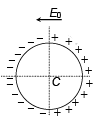The dipole moment of ring is

Solution:
QUESTION: 10

A uniform ring of mass m and radius R can rotate freely about an axis passing through centre C and perpendicular to plane of paper. Half of ring is positively charge and other half is negatively charge. Uniform electric field E0 is switched on along –ve x-axis (Axis are shown in figure) [magnitude of charge density λ]The equilibrium of ring is

Solution:
QUESTION: 11

A uniform ring of mass m and radius R can rotate freely about an axis passing through centre C and perpendicular to plane of paper. Half of ring is positively charge and other half is negatively charge. Uniform electric field E0 is switched on along –ve x-axis (Axis are shown in figure) [magnitude of charge density λ]If ring is slightly disturb from given position, find the angular speed of ring when it rotate by π/2.

Solution:
QUESTION: 12

This section contains 2 questions. Each question contains statements given in two columns, which have to be matched. The statements in Column I are labelled A, B, C and D, while the statements in Column II are labelled p, q, r, s and t. Any given statement in Column I can have correct matching with ONE OR MORE statement(s) in Column II. The appropriate bubbles corresponding to the answers to these questions have to be darkened as illustrated in the following example: If the correct matches are A – p, s and t; B – q and r; C – p and q; and D – s and t; then the correct darkening of bubbles will look like the following:

Three sound sources of same frequency f are arranged as shown in figure. S2 moves towards left
with speed v /n, S3 moves on a circular path of radius R with constant speed 2v/n
, and S1 performs S.H.M. with frequency 100v/nπ s-1and amplitude 1 cm. (n > 12 and v is speed of sound)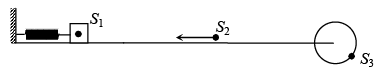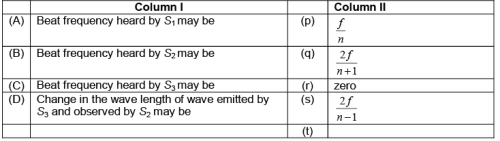Solution:
QUESTION: 13

This section contains 2 questions. Each question contains statements given in two columns, which have to be matched. The statements in Column I are labelled A, B, C and D, while the statements in Column II are labelled p, q, r, s and t. Any given statement in Column I can have correct matching with ONE OR MORE statement(s) in Column II. The appropriate bubbles corresponding to the answers to these questions have to be darkened as illustrated in the following example: If the correct matches are A – p, s and t; B – q and r; C – p and q; and D – s and t; then the correct darkening of bubbles will look like the following: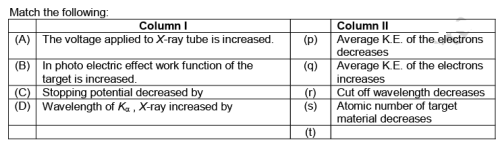Solution:
*Answer can only contain numeric values
QUESTION: 14

This section contains 5 questions. Each question, when worked out will result in one integer from 0 to 9
(both inclusive).

A particle starts moving with velocity 10 m/s in a straight line under an acceleration varying linearly with time. Its velocity time graph is as shown in figure. Its velocity is maximum at t = 3 sec. Find the time (in sec) when the particle stops (tan 370 = ¾)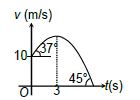Solution:
*Answer can only contain numeric values
QUESTION: 15

A block of mass 1 kg start moving at t = 0 with speed 2 m/s on rough horizontal surface with coefficient of friction 0.2. A horizontal force F is applied in the direction of velocity which varies with time shown in figure (B). Find the speed (in m/s) of particle at t = 3 s (g = 10 m/s2)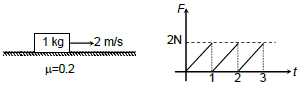Solution:
*Answer can only contain numeric values
QUESTION: 16

One end of a massless rope, which passes over a massless and frictionless pulley P is tied to a hook C while the other end is free. Maximum tension that the rope can bear is 360 N with what value of minimum safe acceleration (in ms–2) can a monkey of 60 kg move down on the rope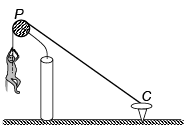Solution:
*Answer can only contain numeric values
QUESTION: 17

Two sources of emf 6V and internal resistance 3Ω and 2Ω are connected to an external resistance R as shown. If potential difference across battery A is zero, then find the value of R (in ohm)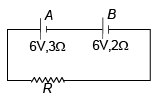Solution:
QUESTION: 18

This section contains 4 multiple choice questions numbered 1 to 4. Each question has 4 choices (A), (B),
(C) and (D), out of which ONLY ONE is correct

The formula of the following chain silicate (pyroxene) can be represented as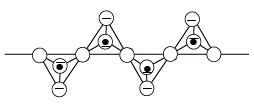Solution:

It is a chain silicate.

QUESTION: 19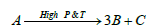is a first order reaction. If A is strong mono protic acid and B is strong diprotic acid and reaction kinetic is studied by using a standard solution of NaOH required toneutralise reaction mixture at different instants of time. When initially we had taken sameconcentration of A in each case.Now it was observer that volume required after 971 sec was double that required initially, then thehalf life of reaction is:

Solution: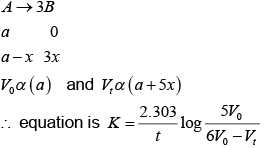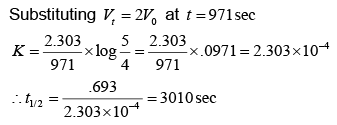QUESTION: 20

Reduction potentials of some ions are given below. Arrange them in decreasing order of oxidizing power.

Ion                                           ClO4−     ​IO4​        BrO4

Reduction potential E/VE= 1.19VE= 1.65VE= 1.74V

Solution:

The reduction potential of the substance is the ability of the substance to be reduced.So as the reduction potential increases reducing ability of the  substance increases.It means oxidizing power of the substance (The ability of the substance to make the other substance to lose the electrons increases which noting but oxidizing power) increases.

So the decreasing order of oxidizing power is BrO4​​ > ​IO4​  > ClO4−

QUESTION: 21

Compound A and B, both were treated with NaOH , producing a single compound C: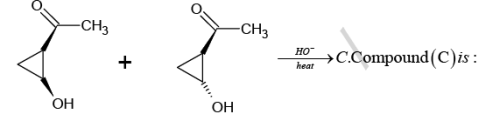Solution: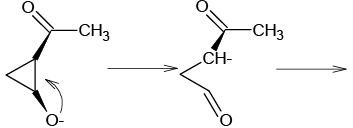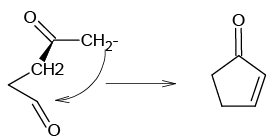QUESTION: 22

This section contains 2 multiple choice questions. Each question has 4 choices (A), (B), (C) and (D) for its answer, out of which ONE OR MORE is/are correct

For the reaction NH3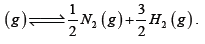. If Kp of the above reaction is 78.1 at 4000Cwhich is not correct:

Solution: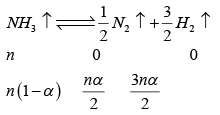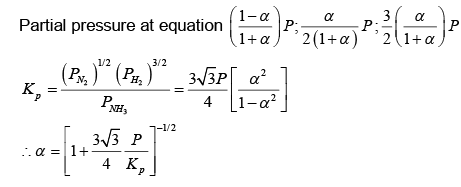*Multiple options can be correct
QUESTION: 23

20 mL of KOH solution was titrated with 0.20 mol L-1 H2SO4 solution in a conductively cell. The data obtained were plotted to give the graph shown below: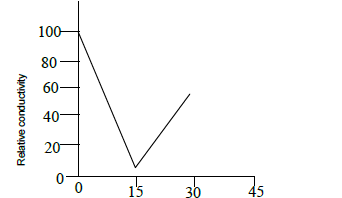The concentration of the KOH solution was:

Solution:

Volume of H2SO4 used at equivalence point is 15mL.

15 x 0.2 x 2 = 20 x conc. Conc. = 0.3

QUESTION: 24

This section contains 2 paragraphs. Based upon one of paragraph 2 multiple choice questions and based on the other paragraphs 3 multiple choice questions have to be answered. Each of these questions has four choices (A), (B), (C) and (D) out of which ONLY ONE is correct

Paragraph for Question Nos. 10 and 11

The Ellingham diagram for the reduction of haematite and that of cuprous oxide given below;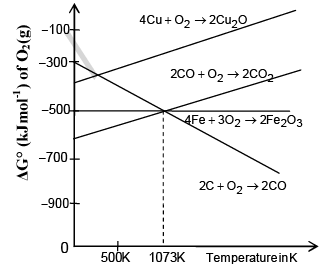On the basis of the above plot, answer the following two questions:

Choose the incorrect statement

Solution:
QUESTION: 25

The Ellingham diagram for the reduction of haematite and that of cuprous oxide given below;On the basis of the above plot, answer the following two questions:

The incorrect statement amongst the following is

Solution:
QUESTION: 26

Paragraph for Question Nos. 12 and 14

Dolacaine, a local anesthetic, is a compound with molecular formula, C13 H20 O2 N2 . It is insoluble in water and dilute NaOH, but soluble in dil. HCl. Upon treatment with NaNO2 and HCl and then withβ -naphthol, a highly coloured solid is formed. When Novocaine is boiled with aqueous NaOH , it slowly dissolves. The alkaline solution is shaken with ether and layers are separated.

Acidification of the aqueous layer causes the precipitation of white solid ‘A’; continued addition of acid causes ‘A’ to redissolve. Upon isolation ‘A’ is found to have melting point of 185 -186° C and the formula C7 H7 O2 N : Evaporation of ether layer leaves a liquid ‘B’ of formula C6 H15 ON. with acetic anhydride gives 'C' C8 H17 O2   N which is insoluble in water and dilute bases, but soluble in dilute HCl. ‘B’ is found to be identical to the compound formed by the action of diethylamine on ethylene oxide.

When compound ‘B’ is treated with p – nitrobenzoyl chloride followed by moderate reduction with Ni/H2 ; the compound formed is

Solution:
QUESTION: 27

Dolacaine, a local anesthetic, is a compound with molecular formula, C13 H20 O2 N2 . It is insoluble in water and dilute NaOH, but soluble in dil. HCl. Upon treatment with NaNO2 and HCl and then withβ -naphthol, a highly coloured solid is formed. When Novocaine is boiled with aqueous NaOH , it slowly dissolves. The alkaline solution is shaken with ether and layers are separated.

Acidification of the aqueous layer causes the precipitation of white solid ‘A’; continued addition of acid causes ‘A’ to redissolve. Upon isolation ‘A’ is found to have melting point of 185 -186° C and the formula C7 H7 O2 N : Evaporation of ether layer leaves a liquid ‘B’ of formula C6 H15 ON. with acetic anhydride gives 'C' C8 H17 O2   N which is insoluble in water and dilute bases, but soluble in dilute HCl. ‘B’ is found to be identical to the compound formed by the action of diethylamine on ethylene oxide.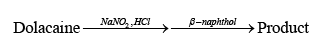Solution:
QUESTION: 28

Dolacaine, a local anesthetic, is a compound with molecular formula, C13 H20 O2 N2 . It is insoluble in water and dilute NaOH, but soluble in dil. HCl. Upon treatment with NaNO2 and HCl and then withβ -naphthol, a highly coloured solid is formed. When Novocaine is boiled with aqueous NaOH , it slowly dissolves. The alkaline solution is shaken with ether and layers are separated.

Acidification of the aqueous layer causes the precipitation of white solid ‘A’; continued addition of acid causes ‘A’ to redissolve. Upon isolation ‘A’ is found to have melting point of 185 -186° C and the formula C7 H7 O2 N : Evaporation of ether layer leaves a liquid ‘B’ of formula C6 H15 ON. with acetic anhydride gives 'C' C8 H17 O2   N which is insoluble in water and dilute bases, but soluble in dilute HCl. ‘B’ is found to be identical to the compound formed by the action of diethylamine on ethylene oxide.

Compound B is having:

Solution:
QUESTION: 29

This section contains 2 questions. Each question contains statements given in two columns, which have to be matched. The statements in Column I are labelled A, B, C and D, while the statements in Column II are labelled p, q, r, s and t. Any given statement in Column I can have correct matching with ONE OR MORE statement(s) in Column II. The appropriate bubbles corresponding to the answers to these questions have to be darkened as illustrated in the following example:

If the correct matches are A – p, s and t; B – q and r; C – p and q; and D – s and t; then the correct darkening of bubbles will look like the following: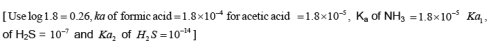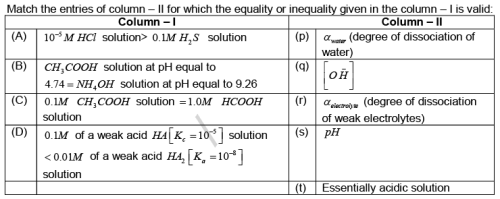Solution:
QUESTION: 30

This section contains 2 questions. Each question contains statements given in two columns, which have to be matched. The statements in Column I are labelled A, B, C and D, while the statements in Column II are labelled p, q, r, s and t. Any given statement in Column I can have correct matching with ONE OR MORE statement(s) in Column II. The appropriate bubbles corresponding to the answers to these questions have to be darkened as illustrated in the following example:

If the correct matches are A – p, s and t; B – q and r; C – p and q; and D – s and t; then the correct darkening of bubbles will look like the following: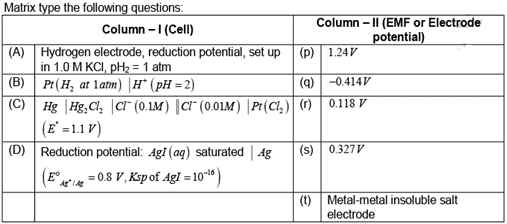Solution: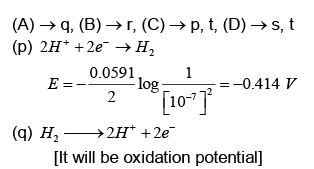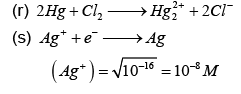*Answer can only contain numeric values
QUESTION: 31

This section contains 5 questions. Each question, when worked out will result in one integer from 0 to 9 (both inclusive).

Sulphide ion react with Na2[Fe(NO)(CN)5] to form a purple coloured compound Na4[Fe(CN)5NOS]. In this reaction the change in oxidation state of iron is?

Solution:
*Answer can only contain numeric values
QUESTION: 32

Gold number of starch is 25. The amount of 0.3125 x a gm of starch is required to prevent
coagulation of 100 ml of gold sol adding 10 ml of 10% NaCl solution? Value of a is………

Solution:
*Answer can only contain numeric values
QUESTION: 33

A coordination compound has empirical formula PdF3 . What is oxidation number of central atom?

Solution: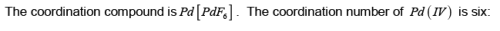*Answer can only contain numeric values
QUESTION: 34

Dimethyl gloyoxime is added to aqueous solution of nickel chloride, made alkaline, by adding NH4 OH solution, a brilliant red ppt is obtained. The number of Ni - N bonds in red ppt is…………..?

Solution:
QUESTION: 35

This section contains 6 multiple choice questions numbered 1 to 6. Each question has 4 choices (A), (B), (C) and (D), out of which ONLY ONE is correct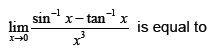Solution:

Use expansion of sin-1 x&tan-1 x to get the result

QUESTION: 36

Area of the region bounded by the curve y=1/x + 1 , the tangent at P (2, 3/2) and the line x = 1 is

Solution:

Plot the curve y - 1 = 1/x  and translate the axis to get required area.

QUESTION: 37

Solution of the differential equation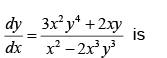Solution: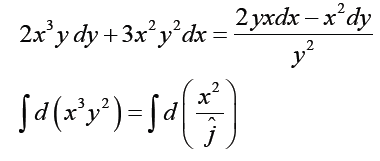QUESTION: 38

Angle between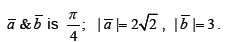Length of longer diagonal of the parallelogram  Length of longer diagonal of the parallelogram having adjacent sides represented by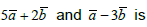Solution: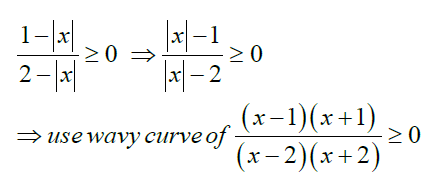QUESTION: 39

A number is selected at random from the set of natural numbers. The probability that the sum of the digits of its square is 39 is

Solution:
QUESTION: 40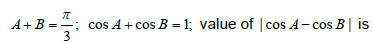Solution:
*Multiple options can be correct
QUESTION: 41

This section contains 3 multiple choice questions. Each question has 4 choices (A), (B), (C) and (D) for its answer, out of which ONE OR MORE is/are correct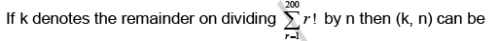Solution:
*Multiple options can be correct
QUESTION: 42

The square roots of the matrix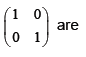Solution: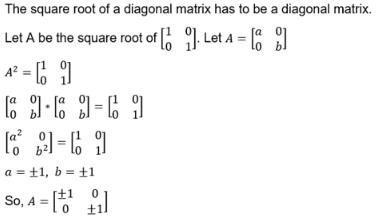QUESTION: 43

Range of the function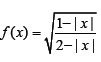contains

Solution:
QUESTION: 44

This section contains 2 paragraphs. Based upon one of paragraph 2 multiple choice questions and based on the other paragraphs 3 multiple choice questions have to be answered. Each of these questions has four choices (A), (B), (C) and (D) out of which ONLY ONE is correct

Paragraph for Question Nos. 10 and 11

a, b, c, d is an A.P. with integer terms and d = a2 + b2 + c2

The common difference is

Solution: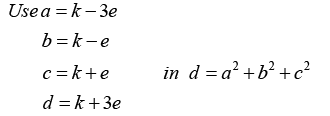QUESTION: 45

a, b, c, d is an A.P. with integer terms and d = a2 + b2 + c2The value of a + 2b + 3c + 4d is

Solution:QUESTION: 46

Paragraph for Question Nos. 12 and 14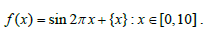Number of points where f achieves local maximum is

Solution:

plot the graphs using periodicity

QUESTION: 47

Paragraph for Question Nos. 12 and 14Number of roots of f (x)  0 in (0, 10) is

Solution:

plot the graphs using periodicity

QUESTION: 48

Paragraph for Question Nos. 12 and 14Number of points where f achieves local minima is

Solution:

plot the graphs using periodicity

QUESTION: 49

This section contains 2 questions. Each question contains statements given in two columns, which have to be matched. The statements in Column I are labelled A, B, C and D, while the statements in Column II are labelled p, q, r, s and t. Any given statement in Column I can have correct matching with ONE OR MORE statement(s) in Column II. The appropriate bubbles corresponding to the answers to these questions have to be darkened as illustrated in the following example:
If the correct matches are A – p, s and t; B – q and r; C – p and q; and D – s and t; then the correct darkening of bubbles will look like the following: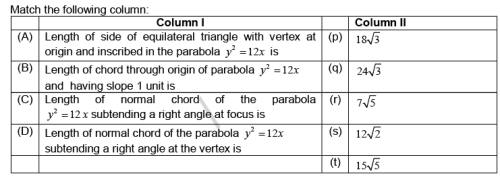Solution:
QUESTION: 50

This section contains 2 questions. Each question contains statements given in two columns, which have to be matched. The statements in Column I are labelled A, B, C and D, while the statements in Column II are labelled p, q, r, s and t. Any given statement in Column I can have correct matching with ONE OR MORE statement(s) in Column II. The appropriate bubbles corresponding to the answers to these questions have to be darkened as illustrated in the following example:
If the correct matches are A – p, s and t; B – q and r; C – p and q; and D – s and t; then the correct darkening of bubbles will look like the following:

Consider the family of lines ax + by + c = 0 where 4a2 + 9b2 - c2 +12ab = 0. Let ‘p’ denote distance of R(6, 8) from the line ax + by + c = 0
Match the following columns: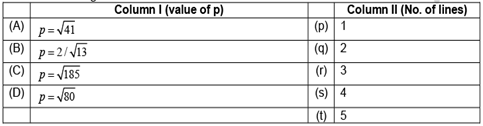Solution: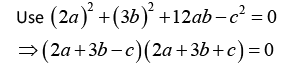*Answer can only contain numeric values
QUESTION: 51

This section contains 5 questions. Each question, when worked out will result in one integer from 0 to 9 (both inclusive).

Number of points of discontinuity of the function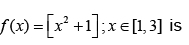Solution:
QUESTION: 52

A tangent to ellipse x2 + 4y2 = 4 meets the ellipse x2 + 2y2 = 6 at P and Q. Tangents at Pand Qare inclined at an angle .π/kThe value of k is

Solution:

use director circle concept

*Answer can only contain numeric values
QUESTION: 53

If n2 + 96 : n∈N, is square of an integer then number of possible values of n is

Solution: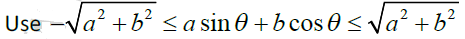*Answer can only contain numeric values
QUESTION: 54

If 2 cosθ + sinθ = 1, then maximum value of 7 cosθ + 6 sinθ is

Solution: